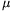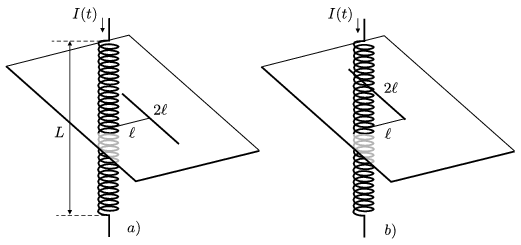Mathematical and Physical Journal
for High Schools
Issued by the MATFUND Foundation
 Already signed up? New to KöMaL?

#Problem P. 4243. (March 2010)

P. 4243. The number of turns of a solenoid (straight coil) of length L=0,5 m is N=2000, the area of its cross section is A=16 cm2, and it has an iron core of relative permittivity ofr=2500. At a moment the current in the coil changes at a rate of 100 A/s. What is the induced voltage in that long straight wire of length 2=20 cm (the wire is next to the centre of the coil)

a) which is perpendicular to the axis of the coil, if its two ends are equidistant from the coil, and its centre is at a distance offrom the coil?

b) which has the same direction as the previous one, and one of its end is at a distance offrom the axis of the coil?(5 pont)

Deadline expired on April 12, 2010.

Sorry, the solution is available only in Hungarian. Google translation

Megoldás. $\displaystyle a)$ $\displaystyle U_a=\frac{1}{4}\mu_0 \mu_r\frac{NA}{L}\frac{\Delta I}{\Delta t}=0,\!50$ V,

$\displaystyle b)$ $\displaystyle U_b=\frac{\arctg 2}{2\pi}\mu_0 \mu_r\frac{NA}{L}\frac{\Delta I}{\Delta t}=0,\!35$ V.

### Statistics:

 22 students sent a solution. 5 points: Balogh Gábor, Béres Bertold, Börcsök Bence, Fonai Dániel, Galzó Ákos Ferenc, Jéhn Zoltán, Kaposvári István, Kószó Simon, Kovács Attila, Kungl Ákos Ferenc, Lájer Márton, Mayer Martin János, Pálovics Péter, Patartics Bálint, Vuchetich Bálint. 4 points: Bodosi Eszter, Filep Gábor, Laczkó Zoltán Balázs, Nagy 111 Miklós, Para Attila. 2 points: 2 students.

Problems in Physics of KöMaL, March 2010